# Topic: Integrals (Test 1)

Topic: Integrals
Q.1
If f (x) be a function such thatthen f (x) is equal to
A.  + C
B.  + C
C.  + C
D. x log (x e) + C
Answer : Option A
Explaination / Solution:

Integrating both sides, we get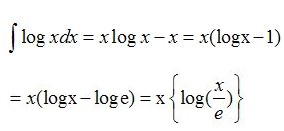Workspace
Report
Q.2
is equal to
A. f(x) + C
B. log ( f(x) ) + C
C.  + C
D. 2√f(x) + C
Answer : Option D
Explaination / Solution:

Workspace
Report
Q.3
dx is equal to
A. 12log(2x)+C
B. log 2x + C
C. (x−1)log(1−x)+1−xlnx+C
D. (3x+1)log(2+x)+1−xln2x+C
Answer : Option C
Explaination / Solution:

log(1x)dxlogx dx(x1)log(1x)+1xlnx+C
Workspace
Report
Q.4
dx is equal to
A. x log (log x)  + C
B. xlog2x1x+2+C
C. x log (log x) +  + C
D. x log (log x) + C
Answer : Option D
Explaination / Solution:Workspace
Report
Q.5
Which of the following is not equal to
A.  + C
B. - + C
C. sin1x+C
D.  (secx)(cos1x)(cosx)+C
Answer : Option B
Explaination / Solution:Workspace
Report
Q.6

dx is equal to

A. 3cot2x3+C
B. cotx2+C
C. tanx2+C
D. 12tanx+C
Answer : Option C
Explaination / Solution:Workspace
Report
Q.7
is equal to
A.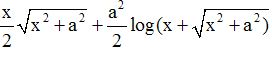Standard Formulae  Can be done by By-Part taking '1' as the second function and
as first function.
B. tan1(x2+x+2)+C
C.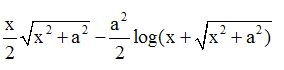D.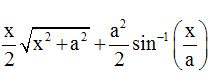Answer : Option A
Explaination / Solution: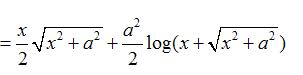Workspace
Report
Q.8
cosx(1+sinx)(2+sinx)dx=
A. log(2sinx1sinx)+C
B. log (1-sin x).(2-sin x) + C
C. log(2+sinx1sin2x)+C
D. log(1+sinx2+sinx)+C
Answer : Option D
Explaination / Solution:Workspace
Report
Q.9
is equal to
A. ex+f(x)+C
B. ef(x)+f(ex)+C
C. ex(f(x)f(x))+C
D. exf(x)+C
Answer : Option D
Explaination / Solution:Workspace
Report
Q.10
∫ |x| dx
A. 2x23+C
B. 1
C. (12)x|x|+C
D.  + C
Answer : Option C
Explaination / Solution:Workspace
Report